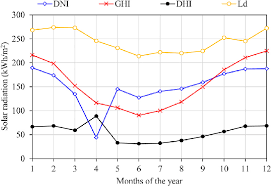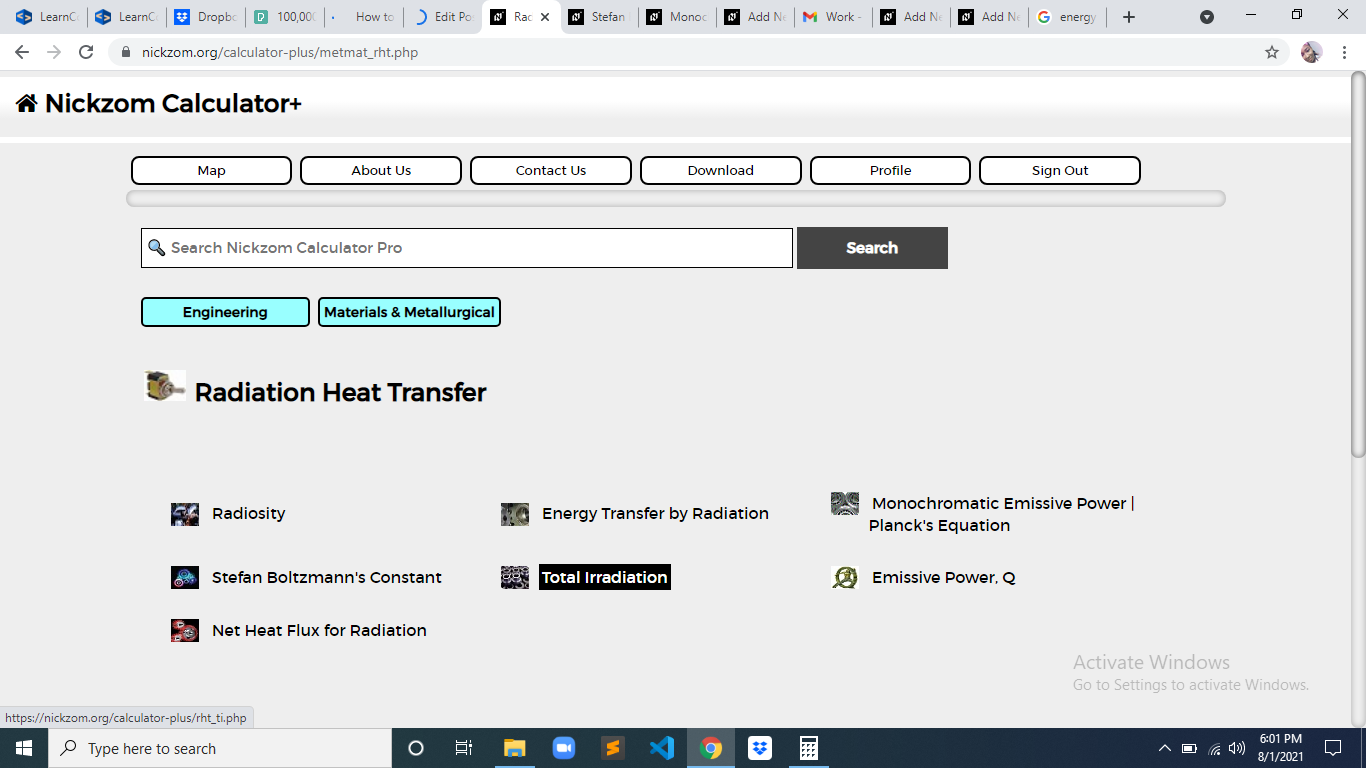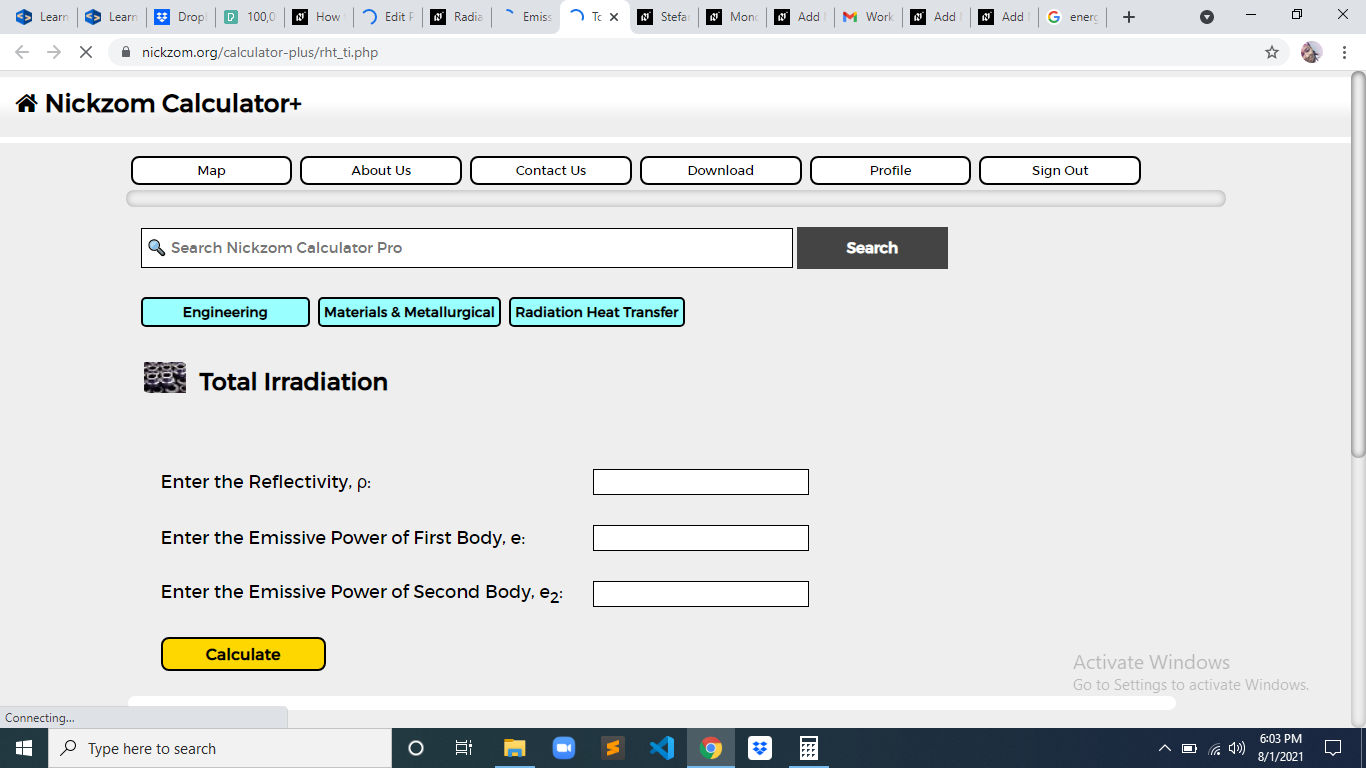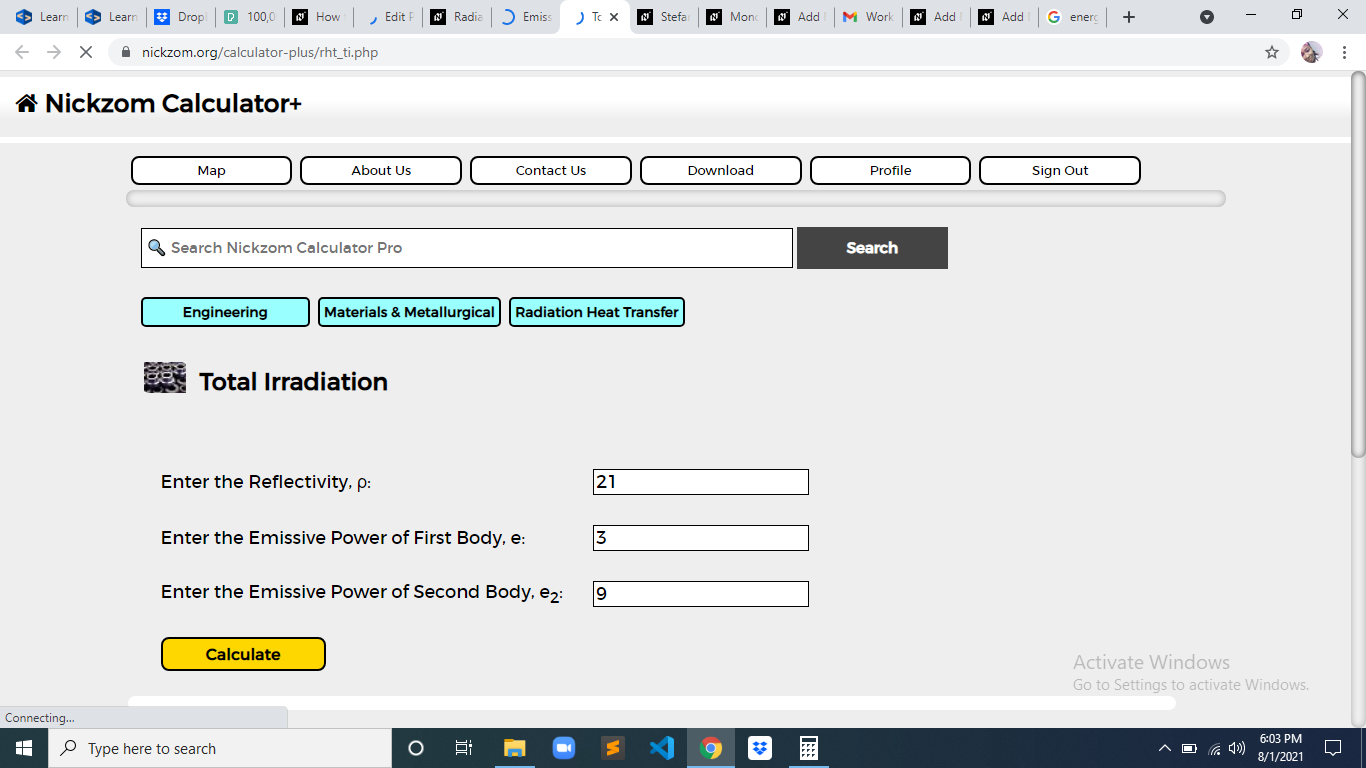# How to Calculate and Solve for Total Irradiation | Radiation Heat TransferThe image above represents total irradiation.

To compute for total irradiation, three essential parameters are needed and these parameters are Reflectivity (ρ), Emissive Power of First Body (e) and Emissive Power of Second Body (e2).

The formula for calculating total irradiation:

G = e2 + ρe / 1 – ρ

Where:

ρ = Reflectivity
e = Emissive Power of First Body
e2 = Emissive Power of Second Body

Let’s solve an example;
Given that the reflectivity is 21, the emissive power of first body is 3 and the emissive power of second body is 9. Find the total irradiation?

This implies that;

ρ = Reflectivity = 21
e = Emissive Power of First Body = 3
e2 = Emissive Power of Second Body = 9

G = e2 + ρe / 1 – ρ
G = 9 + 21(3) / 1 – 21
G = 9 + 63 / -20
G = 72 / -20
G = -3.6

Therefore, the total irradiation is -3.6.

Nickzom Calculator – The Calculator Encyclopedia is capable of calculating the total irradiation.

To get the answer and workings of the total irradiation using the Nickzom Calculator – The Calculator Encyclopedia. First, you need to obtain the app.

You can get this app via any of these means:

To get access to the professional version via web, you need to register and subscribe for NGN 2,000 per annum to have utter access to all functionalities.
You can also try the demo version via https://www.nickzom.org/calculator

Apple (Paid) – https://itunes.apple.com/us/app/nickzom-calculator/id1331162702?mt=8
Once, you have obtained the calculator encyclopedia app, proceed to the Calculator Map, then click on Materials and Metallurgical under Engineering.Now, Click on Radiation Heat Transfer under Materials and MetallurgicalThe screenshot below displays the page or activity to enter your values, to get the answer for the total irradiation according to the respective parameter which is the Reflectivity (ρ), Emissive Power of First Body (e) and Emissive Power of Second Body (e2).Now, enter the values appropriately and accordingly for the parameters as required by the Reflectivity (ρ) is 21, Emissive Power of First Body (e) is 3 and Emissive Power of Second Body (e2) is 9.Finally, Click on CalculateAs you can see from the screenshot above, Nickzom Calculator– The Calculator Encyclopedia solves for the total irradiation and presents the formula, workings and steps too.